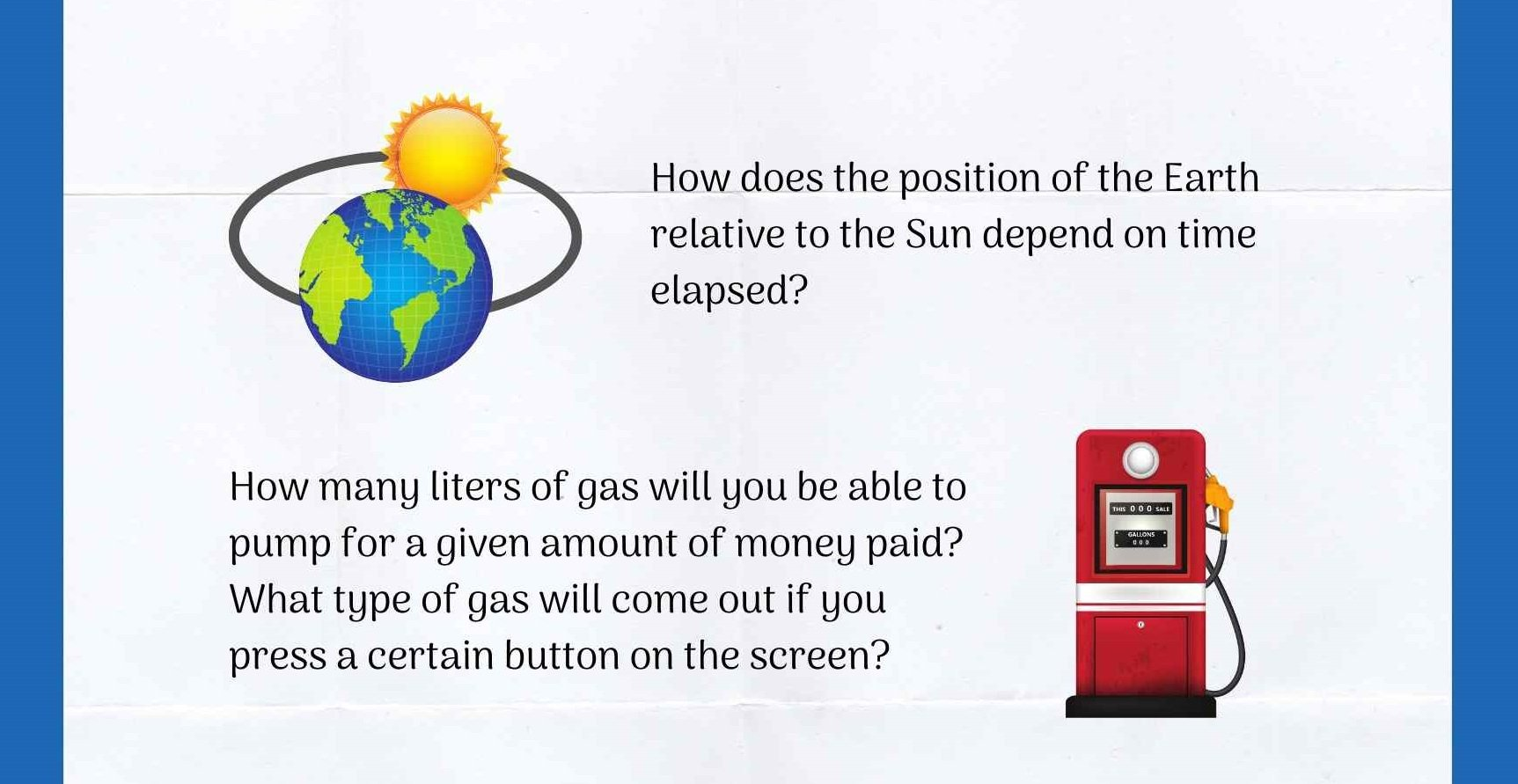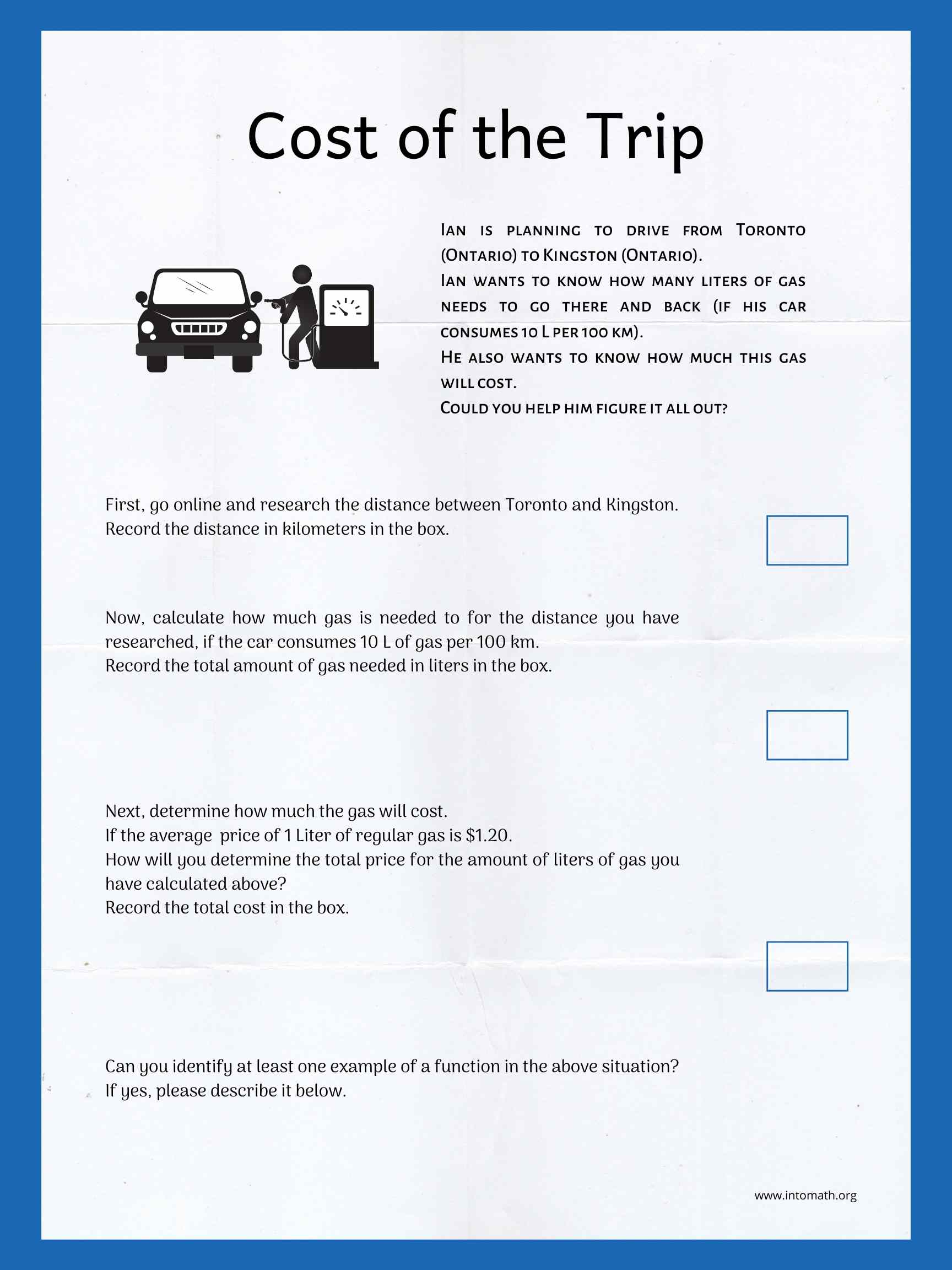# What is a function and how to introduce itA concept of a function is being officially introduced in different grades in different countries.  In Canada, teachers usually start calling a particular relation a function around grades 9 – 10. Teachers in the USA usually introduce it around grades 8 – 9. In a number of European countries the term is already used widely by as early as grade 7.

There really is no right time to start using the term and labeling it as such. However, when students are already dealing with the concept in their math class and are able to comprehend its application in the real world, why not introduce the terminology and let them get used to it?

#### What is a function?

It is a relationship between the independent and the dependent quantity, where for one input there is only one possible output. The output is essentially a function of the input defined by a set of ordered pairs (x, y). All possible x-values define the domain. All possible y-values define the range. Some points on the graph representing a function are called “critical points”.

The most basic example of function is a linear function.

There are examples of a linear function all around us and they can be used to illustrate the concept to our students.

We do not even need to immediately identify the relation type when we first introduce it.

The main goal initially is to get students to understand how the relationship within a function works.#### Real life situation

Once students have been exposed to a number of examples and have grasped the idea, they can try to independently work with the concept. They can be presented with a real life situation. And discover how a particular relation is actually a function.

Here is an example of a situation where students can investigate the application of a function.#### Equation and graph

Next, students can be introduced to the concept of a linear function and make a connection between the equation and its word representation. This helps students develop an understanding of how words can be translated into algebraic expressions and equations.  In addition, they can then graph the relation using a table of ordered pairs of independent/dependent variables.#### Domain and range

One important aspect that cannot be overlooked when introducing and discussing the concept is which sets of inputs and outputs are possible for any given function – domain and range. Can time be negative? Does a ball reach a maximum height after it was hit and before falling back to the ground?

Here is an example of how based on a real life example students can investigate the possible restrictions on the independent and the dependent variables.Browse IntoMath for more resources such as video lessons, ready-to-go notes and practice worksheets on a variety of topics.

0